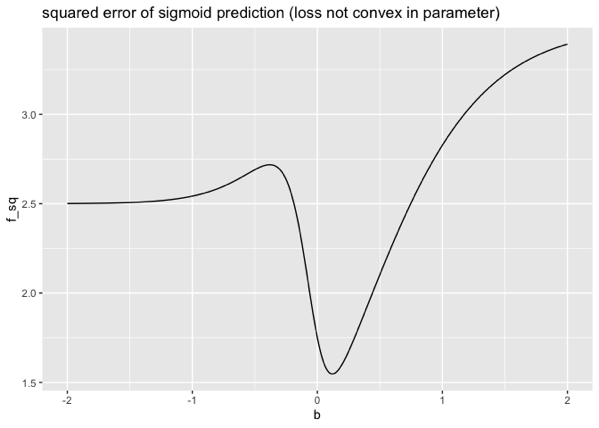# An Example Where Square Loss of a Sigmoid Prediction is not Convex in the Parameters

I’ve added a worked `R` example of the non-convexity, with respect to model parameters, of square loss of a sigmoid-derived prediction here.This is finishing an example for our `Python` note “Why not Square Error for Classification?”. Reading that note will give a usable context and background for this diagram.

The undesirable property is: such a graph says that a parameter value of `b = -1` and `b = -0.25` have similar losses, but parameters values in-between are worse. This might seem paradoxical, but it is an artifiact of the loss-function – not an actual property of the data or model. The same note shows the deviance loss has the desirable convex property: interpolations of good parameter values are also good.

Categories: Mathematics Opinion Tutorials### jmount

Data Scientist and trainer at Win Vector LLC. One of the authors of Practical Data Science with R.